# Noise: Input vs Output Referred Noise

Sep 23, 2012

## Introduction

The total noise of a typical op-amp circuit is composed of thermal noise due to external resistances, op-amp voltage noise and op-amp current noise flowing through external resistors. These different noise components in general will experience different noise gains at different frequencies depending on the circuit components and of course will be naturally filtered by the circuits bandwidth response. Circuit noise is often specified as an input referred rms spectral noise density per root unit bandwidth where the total output noise is simply the input referred noise spectral density multiplied by the circuit gain and the square root of the "noise bandwidth". However, in some circuits, such as the common transimpedance circuit (Tz) , it is not possible to simply factor out a single "gain" or "bandwidth" as different noise sources experience different gain profiles as a function of frequency. Most notably, the op-amp voltage noise in Tz circuits will experience a voltage-gain increase due to a zero formed by the capacitance Ci at the inverting input breaking with the feedback resistance Rf. This means that the amplified voltage noise in Tz circuits often dominates the total noise. In this case it is more useful to specify the total output-referred noise.

The purpose of this note is to show how the total output noise in the general case reduces to the well-known input-referred noise expression for cases where the circuit "noise gain" is fairly constant with frequency over the circuits response bandwidth. This will be the case with most standard voltage-gain amplifier configurations.

## The General Case: Output Referred Noise

For noise discussion purposes, consider the fairly general op-amp configuration shown below. (We have not included a Cp capacitance at the non-inverting input for simplicity.). This configuration applies to inverting and non-inverting voltage amplifier configurations as well as transimpedance configurations:Assuming constant spectral densities en and in and a single-pole model for the op-amp open-loop gain profile, the exact total output-referred noise in Volts, integrated over all frequency, is given by:where en and in are the op-amp voltage and current noise spectral densities respectively, GBW is the op-amp gain-bandwidth product, BW is the basic bandwidth parameter, N0 is the DC noise gain, F0 is the undamped resonant frequency, Q is the quality factor parameter, and Fz and Fp are the noise-gain zero and pole frequencies: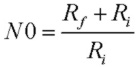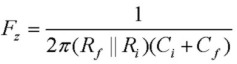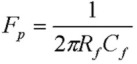Or we can identify noise bandwidth parameters NBWen and NBWin_th which as can be seen are different for the two different groups of noise sources:where the NBWin_th term is:Note that the NBWen term is greater by a factor of 1+BW/Fz which tends to make the noise contribution from en and noise sources at the non-inverting input associated with Rp dominant, if BW/Fz >>1. This is often the case for transimpedance circuits where the zero frequency Fz is considerably lower than the circuit response bandwidth because Ri is usually much higher than Rf and Ci is often considerable. Therefore in general, a single "noise bandwidth" cannot be factored out.

## Special Case: Input Referred Noise

To see how the general expression above for output-referred total noise reduces to the familiar result for input-referred noise, consider the case where BW/Fz <<1. This will often apply for voltage amplifier circuits where the low value of Ri moves Fz out to high frequency. This means that the noise-gain function is fairly flat at the low-frequency value of N0 over the entire response bandwidth of the circuit. In this limit it is seen that the two noise bandwidths are identical and equal to NBWin_th. In this case we can factor out this noise bandwidth and also factoring out the noise gain N0: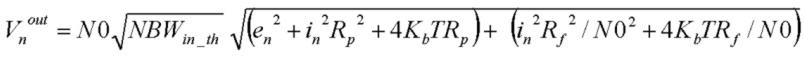and substituting for N0 under the radical and grouping terms together, we recognize a familiar result: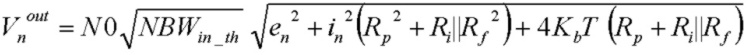where Ri||Rf is the parallel combination of Ri and Rf. We now identify the total output-referred noise (in Volts) as an input referred rms noise density Vnin (in Volts/√Hz) multiplied by the square root of a noise bandwidth and the constant noise gain N0: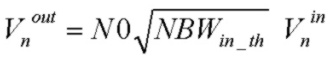where:What is the value of the noise bandwidth in this case? Referring to the expression above for NBWin_th, we see that the results are again familiar in two common limits: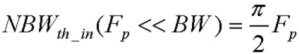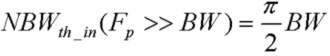exactly as expected for the noise bandwidth of single-pole filters when the circuit response is controlled by either the Fp pole frequency rolloff or the op-amp GBW product limit.